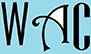## Algebra Question for Title 1 Schools: Work Rate Question?

10) Uyi worked 22 hours this week and made \$132.  If she works 15 hours next week, how much will she make at the same rate?

22 hours  = \$132

1 hour = \$x

Cross multiply to find how much made per hour

22x = 132

you have to make “x” subject of formula by moving “22” to the other side. When you go to the other side, addition becomes subtraction, multiplication becomes division, and vice versa.

x = 132/22 (22 was multiplication at the other end)

x = \$6 per hour

15 hours x 6 = \$90